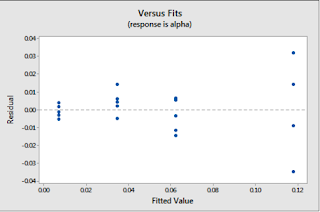## Monday, 18 September 2017

### Assumptions of Linear Regression, Multicollinearity & Outliers Detection

Assumption Of Linear Regression-

1.       Mean of response, at each value of predictor x is Linear function of x.
2.       Error terms should be Independent of each other.
3.       Error terms should be Normally distributed.
4.       Error terms should have Equal variance.

These can be termed as LINE assumptions.

Residuals v/s fitted values graph can be used to test assumptions of linear relationship of Y and x values, Equal variance of error terms.

• The residuals "bounce randomly" around the 0 line. This suggests that the assumption that the relationship is linear is reasonable.
• The residuals roughly form a "horizontal band" around the 0 line. This suggests that the variances of the error terms are equal.
• No one residual "stands out" from the basic random pattern of residuals. This suggests that there are no outliers.

Residuals v/s fitted values graph when relationship is not linear or variance is not constant for error terms.

Relationship is not linear- Most of the values will not lie near y=0, showing deviation from linear relationship. Had it a perfect linear relation all residuals must be 0.

Error terms don’t have constant variance-  As the fitted values are increasing error terms are increasing.Hetroscedastic residuals
• The plot has a "fanning" effect. That is, the residuals are close to 0 for small x values and are more spread out for large x values.
• The plot has a "funneling" effect. That is, the residuals are spread out for small x values and close to 0 for large x values.
• Or, the spread of the residuals in the residuals vs. fits plot varies in some complex fashion.
Residual v/s order plot- Error terms should be independent of each other. There should not be autocorrelation/trend in residuals. If the data are obtained in a time (or space) sequence, a residuals vs. order plot helps to see if there is any correlation between the error terms that are near each other in the sequence.
If we draw sequence of residuals in first residuals /order plot , we get next plot which shows negative auto-correlation. If we have such correlation it’s time to move to time series from regression.

We can simply draw distribution of residuals. A normal distribution has a bell-shaped density curve described by its mean and standard deviation . The density curve is symmetrical, centered about its mean, with its spread determined by its standard deviation. if the residuals follow a normal distribution with mean µ and variance σ2, then a plot of the theoretical percentiles of the normal distribution versus the observed sample percentiles should be approximately linear.

Handling Outliers and Multi-collinearity-

Outliers or influential data points can be identified by Cook’s distance or by Difference in fits. Both use same idea of identifying influential points. They fit the regression without i’th observation and see the change in y values. Higher the change more influential is I th variable.